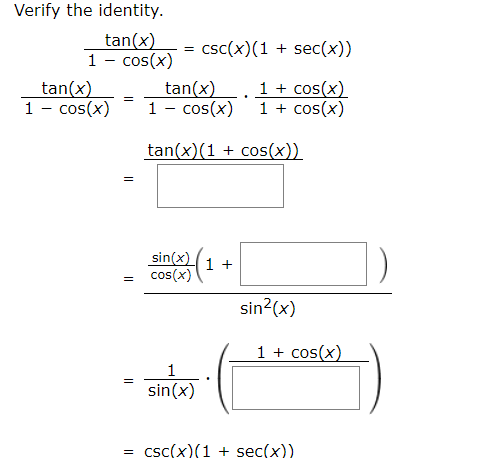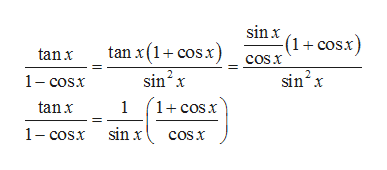# Verify the identitytan(x)Cos(x)csc(x) (1 sec(x))11cos(x)1cos(x)tan(x)cos(x)tan(x)1 - cos(x)tan(x)(1cos(x))=sin(x)cos(x)1 +sin2(x)1cos(x)1=sin(x)= cSc(x)(1 + sec(x))

Question
24 viewshelp_outlineImage TranscriptioncloseVerify the identity tan(x) Cos(x) csc(x) (1 sec(x)) 1 1cos(x) 1cos(x) tan(x) cos(x) tan(x) 1 - cos(x) tan(x)(1cos(x)) = sin(x) cos(x) 1 + sin2(x) 1cos(x) 1 = sin(x) = cSc(x)(1 + sec(x)) fullscreen
check_circle

Step 1

Given identity to be verified is

Step 2

Firstly, we multiply and divide L.H.S by 1+cosx

Step 3

Now , we use identity ...help_outlineImage Transcriptionclosesinx (1+cosx) tan x(1 cos.x) sin2 x 1+cosx tanx cosx sin2x 1-cosx tanx 1 sin x 1- cosx cosx fullscreen

### Want to see the full answer?

See Solution

#### Want to see this answer and more?

Solutions are written by subject experts who are available 24/7. Questions are typically answered within 1 hour.*

See Solution
*Response times may vary by subject and question.
Tagged in

### Other